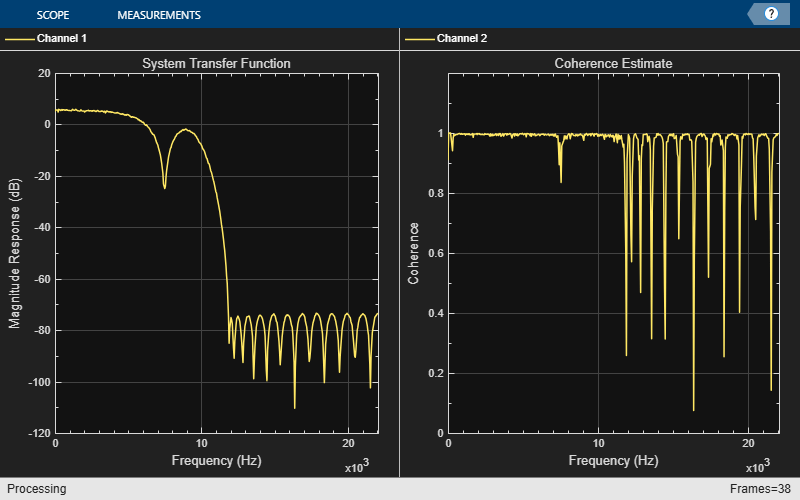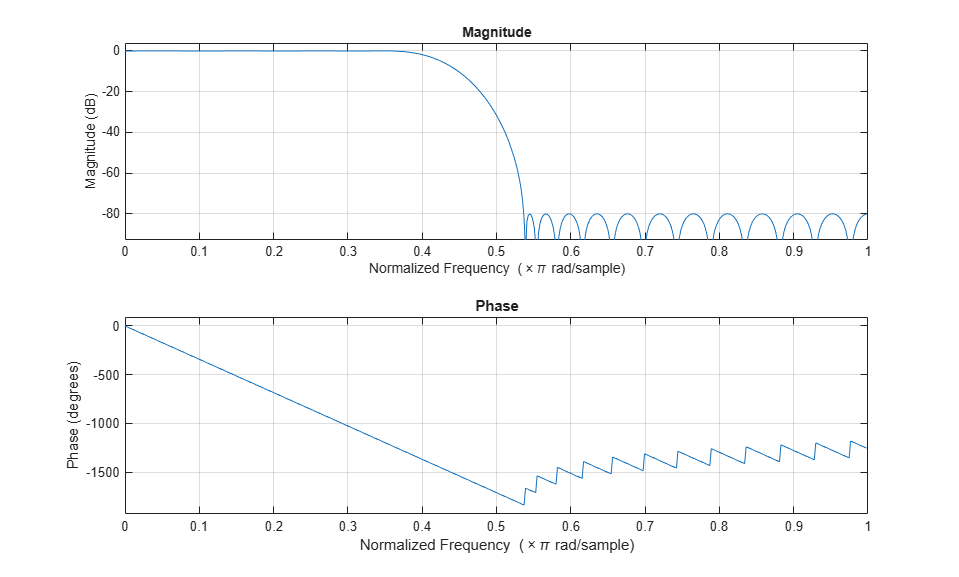## Estimate the Transfer Function of an Unknown System

You can estimate the transfer function of an unknown system based on the system's measured input and output data.

In DSP System Toolbox™, you can estimate the transfer function of a system using the `dsp.TransferFunctionEstimator` System object™ in MATLAB® and the Discrete Transfer Function Estimator block in Simulink®. The relationship between the input x and output y is modeled by the linear, time-invariant transfer function Txy. The transfer function is the ratio of the cross power spectral density of x and y, Pyx, to the power spectral density of x, Pxx:

`${T}_{xy}\left(f\right)=\frac{{P}_{yx}\left(f\right)}{{P}_{xx}\left(f\right)}$`

The `dsp.TransferFunctionEstimator` object and Discrete Transfer Function Estimator block use the Welch’s averaged periodogram method to compute the Pxx and Pxy. For more details on this method, see Spectral Analysis.

Coherence

The coherence, or magnitude-squared coherence, between x and y is defined as:

`${C}_{xy}\left(f\right)=\frac{{|{P}_{xy}|}^{2}}{{P}_{xx}*{P}_{yy}}$`

The coherence function estimates the extent to which you can predict y from x. The value of the coherence is in the range 0 ≤ Cxy(f) ≤ 1. If Cxy = 0, the input x and output y are unrelated. A Cxy value greater than 0 and less than 1 indicates one of the following:

• Measurements are noisy.

• The system is nonlinear.

• Output y is a function of x and other inputs.

The coherence of a linear system represents the fractional part of the output signal power that is produced by the input at that frequency. For a particular frequency, 1 – Cxy is an estimate of the fractional power of the output that the input does not contribute to.

When you set the `OutputCoherence` property of `dsp.TransferFunctionEstimator` to `true`, the object computes the output coherence. In the Discrete Transfer Function Estimator block, to compute the coherence spectrum, select the Output magnitude squared coherence estimate check box.

### Estimate the Transfer Function in MATLAB

To estimate the transfer function of a system in MATLAB™, use the `dsp.TransferFunctionEstimator` System object™. The object implements the Welch's average modified periodogram method and uses the measured input and output data for estimation.

Initialize the System

The system is a cascade of two filter stages: dsp.LowpassFilter and a parallel connection of dsp.AllpassFilter and dsp.AllpoleFilter.

```allpole = dsp.AllpoleFilter; allpass = dsp.AllpassFilter; lpfilter = dsp.LowpassFilter; ```

Specify Signal Source

The input to the system is a sine wave with a frequency of 100 Hz. The sampling frequency is 44.1 kHz.

```sine = dsp.SineWave('Frequency',100,'SampleRate',44100,... 'SamplesPerFrame',1024); ```

Create Transfer Function Estimator

To estimate the transfer function of the system, create the `dsp.TransferFunctionEstimator` System object.

```tfe = dsp.TransferFunctionEstimator('FrequencyRange','onesided',... 'OutputCoherence', true); ```

Create Array Plot

Initialize two `dsp.ArrayPlot` objects: one to display the magnitude response of the system and the other to display the coherence estimate between the input and the output.

```tfeplotter = dsp.ArrayPlot('PlotType','Line',... 'XLabel','Frequency (Hz)',... 'YLabel','Magnitude Response (dB)',... 'YLimits',[-120 20],... 'XOffset',0,... 'XLabel','Frequency (Hz)',... 'Title','System Transfer Function',... 'SampleIncrement',44100/1024); coherenceplotter = dsp.ArrayPlot('PlotType','Line',... 'YLimits',[0 1.2],... 'YLabel','Coherence',... 'XOffset',0,... 'XLabel','Frequency (Hz)',... 'Title','Coherence Estimate',... 'SampleIncrement',44100/1024); ```

By default, the x-axis of the array plot is in samples. To convert this axis into frequency, set the 'SampleIncrement' property of the `dsp.ArrayPlot` object to Fs/1024. In this example, this value is 44100/1024, or 43.0664. For a two-sided spectrum, the `XOffset` property of the `dsp.ArrayPlot` object must be [-Fs/2]. The frequency varies in the range [-Fs/2 Fs/2]. In this example, the array plot shows a one-sided spectrum. Hence, set the `XOffset` to 0. The frequency varies in the range [0 Fs/2].

Estimate the Transfer Function

The transfer function estimator accepts two signals: input to the two-stage filter and output of the two-stage filter. The input to the filter is a sine wave containing additive white Gaussian noise. The noise has a mean of zero and a standard deviation of 0.1. The estimator estimates the transfer function of the two-stage filter. The output of the estimator is the frequency response of the filter, which is complex. To extract the magnitude portion of this complex estimate, use the abs function. To convert the result into dB, apply a conversion factor of 20*log10(magnitude).

```for Iter = 1:1000 input = sine() + .1*randn(1024,1); lpfout = lpfilter(input); allpoleout = allpole(lpfout); allpassout = allpass(lpfout); output = allpoleout + allpassout; [tfeoutput,outputcoh] = tfe(input,output); tfeplotter(20*log10(abs(tfeoutput))); coherenceplotter(outputcoh); end ```The first plot shows the magnitude response of the system. The second plot shows the coherence estimate between the input and output of the system. Coherence in the plot varies in the range [0 1] as expected.

Magnitude Response of the Filter Using fvtool

The filter is a cascade of two filter stages - dsp.LowpassFilter and a parallel connection of dsp.AllpassFilter and dsp.AllpoleFilter. All the filter objects are used in their default state. Using the filter coefficients, derive the system transfer function and plot the frequency response using freqz. Below are the coefficients in the [Num] [Den] format:

• All pole filter - [1 0] [1 0.1]

• All pass filter - [0.5 -1/sqrt(2) 1] [1 -1/sqrt(2) 0.5]

• Lowpass filter - Determine the coefficients using the following commands:

```lpf = dsp.LowpassFilter; Coefficients = coeffs(lpf); ```

Coefficients.Numerator gives the coefficients in an array format. The mathematical derivation of the overall system transfer function is not shown here. Once you derive the transfer function, run fvtool and you can see the frequency response below:The magnitude response that fvtool shows matches the magnitude response that the `dsp.TransferFunctionEstimator` object estimates.

### Estimate the Transfer Function in Simulink

To estimate the transfer function of a system in Simulink, use the Discrete Transfer Function Estimator block. The block implements the Welch's average modified periodogram method and uses the measured input and output data for estimation.

The system is a cascade of two filter stages: a lowpass filter and a parallel connection of an allpole filter and allpass filter. The input to the system is a sine wave containing additive white Gaussian noise. The noise has a mean of zero and a standard deviation of 0.1. The input to the estimator is the system input and the system output. The output of the estimator is the frequency response of the system, which is complex. To extract the magnitude portion of this complex estimate, use the Abs block. To convert the result into dB, the system uses a dB (1 ohm) block.Open and Inspect the Model

To open the model, enter `ex_transfer_function_estimator` in the MATLAB command prompt.

Here are the settings of the blocks in the model.

BlockParameter ChangesPurpose of the block
Sine Wave
• Sample time to `1/44100`

• Samples per frame to `1024`

Sinusoid signal with frequency at 100 Hz

Random Source
• Source type to `Gaussian`

• Variance to 0.01

• Sample time to 1/44100

• Samples per frame to 1024

Random Source block generates a random noise signal with properties specified through the block dialog box
Lowpass FilterNo changeLowpass filter
Allpole FilterNo changeAllpole filter with coefficients ```[1 0.1]```
Discrete Filter
• Numerator to ```[0.5 -1/sqrt(2) 1]```

• Denominator to ```[1 -1/sqrt(2) 0.5]```

Allpass filter with coefficients ```[-1/sqrt(2) 0.5]```
Discrete Transfer Function Estimator
• Frequency range to `One-sided`

• Number of spectral averages to 8

Transfer function estimator
AbsNo changeExtracts the magnitude information from the output of the transfer function estimator
First Array Plot block

Click :

• Select Style and set Plot type to `Line`.

• Select Configuration Properties: From the Main tab, set Sample increment to `44100/1024` and X-offset to `0`. In the Display tab, specify the Title as ```Magnitude Response of the System in dB```, X-label as `Frequency (Hz)`, and Y-label as `Amplitude (dB)`

Shows the magnitude response of the system
Second Array Plot block

Click :

• Select Style and set Plot type to `Line`.

• Select Configuration Properties: From the Main tab, set Sample increment to `44100/1024` and X-offset to `0`. In the Display tab, specify the Title as `Coherence Estimate`, X-label as `Frequency (Hz)`, and Y-label as `Amplitude`

Shows the coherence estimate

By default, the x-axis of the array plot is in samples. To convert this axis into frequency, the Sample increment parameter is set to `Fs/1024`. In this example, this value is `44100/1024`, or `43.0664`. For a two-sided spectrum, the X-offset parameter must be `–Fs/2`. The frequency varies in the range ```[-Fs/2 Fs/2]```. In this example, the array plot shows a one-sided spectrum. Hence, the X-offset is set to 0. The frequency varies in the range `[0 Fs/2]`.

Run the ModelThe first plot shows the magnitude response of the system. The second plot shows the coherence estimate between the input and output of the system. Coherence in the plot varies in the range `[0 1]` as expected.